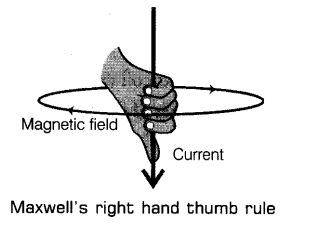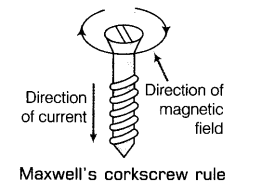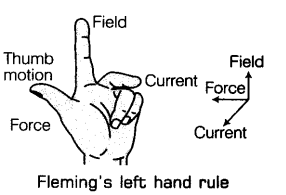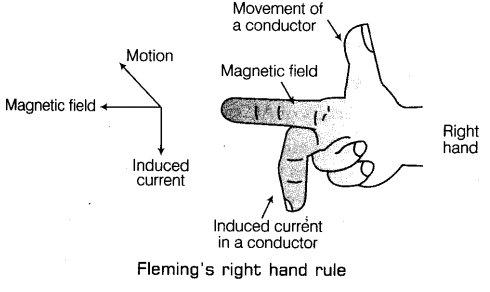# State the rule to determine the direction of a (i) magnetic field produced around a straight conductor carrying-current

State the rule to determine the direction of a
(i) magnetic field produced around a straight conductor carrying-current.
(ii) force experienced by a current-carrying straight conductor placed in a magnetic field.
(iii) current induced in a coil due to its rotation in magnetic field.

(i) Maxwell’s Right Hand Thumb Rule:
The- direction of the magnetic field produced by a current-carrying conductor is given by Maxwell’s right hand thumb rule.It states that if you holding the current-carrying conductor in your right hand such that the thumb points in direction of the current, then the direction in which the fingers encircle, gives the direction of magnetic lines. This rule is also called Maxwell’s Corkscrew Rule.
This rule can also be stated as if we consider ourselves driving a corkscrew in the direction of the current, then the direction in which we rotate the screw is the direction of the magnetic field.(ii) Fleming’s Left Hand Rule:
The direction of force which acts on the current-carrying conductor placed in a magnetic field is given by Fleming’s left hand rule.It states that if the forefinger, thumb and middle finger of left hand are stretched mutually perpendicular and the forefinger points along the direction of external magnetic field, middle finger indicates the direction of current, then thumb points along the direction of force acting on the conductor.

(iii) Fleming’s Right Hand Rule:
The direction of induced current in a straight conductor is given by Fleming’s right hand rule.
It states that if we stretch the thumb, forefinger and the middle finger of right hand at right angles to one another in such a way that the forefinger points in the direction of magnetic field.
Then, thumb gives the direction of motion of conductor (force), forefinger indicates direction of magnetic field and the middle finger points the direction of induced current.